﻿ 基于voronoi图的材料初始组织的生成
«上一篇文章快速检索 高级检索

 哈尔滨工程大学学报2018, Vol. 39Issue (6): 1093-1097  DOI: 10.11990/jheu.2017030080

### 引用本文LIU Yingwei, ZHANG Yang. The generation of the initial materials microstructure based on the voronoi diagram[J]. Journal of Harbin Engineering University, 2018, 39(6), 1093-1097. DOI: 10.11990/jheu.201703008.### 文章历史

The generation of the initial materials microstructure based on the voronoi diagram
LIU Yingwei, ZHANG Yang
Institute for Metallic Materials, College of Materials Science and Chemical Engineering, Harbin Engineering University, Harbin 150001, China
Abstract: When the Monte Carlo method is used to simulate microstructure evolution, defects exist in the initial material microstructure due to traditional methods. Such defects can be overcome by adopting the Voronoi diagram. In this study, a nonrestrictive Voronoi diagram was created by Matlab. The unrestricted Voronoi diagram was cut by angle comparison to generate a restrictive Voronoi diagram conforming to reality. Finally, the strategies of angle comparison and clockwise search were used to group the line segments in the restrictive Voronoi diagram in accordance with the polygons to which the line segments were subordinated. Thus, the initial material microstructure was generated in the tier of data structure. Calculations were carried out by a program prepared based on the abovementioned algorithm. From a statistical perspective, the generated microstructure was very close to the real microstructure, thereby laying a solid foundation for follow-up simulation.
Key words: Monte Carlo    microstructure evolution    voronoi diagram    data structure    homogeneous nucleation    crystallization    grain statistics    normal distribution

1 voronoi图的生成 1.1 非限制性voronoi图

Matlab的MPT工具箱中的voronoi(X, Y)函数有生成voronoi图的功能, 其调用格式为

 $\left[ {{\rm{Vertex\_X,Vertex\_Y}}} \right] = {\rm{voronoi}}\left( {X,Y} \right)$ (1)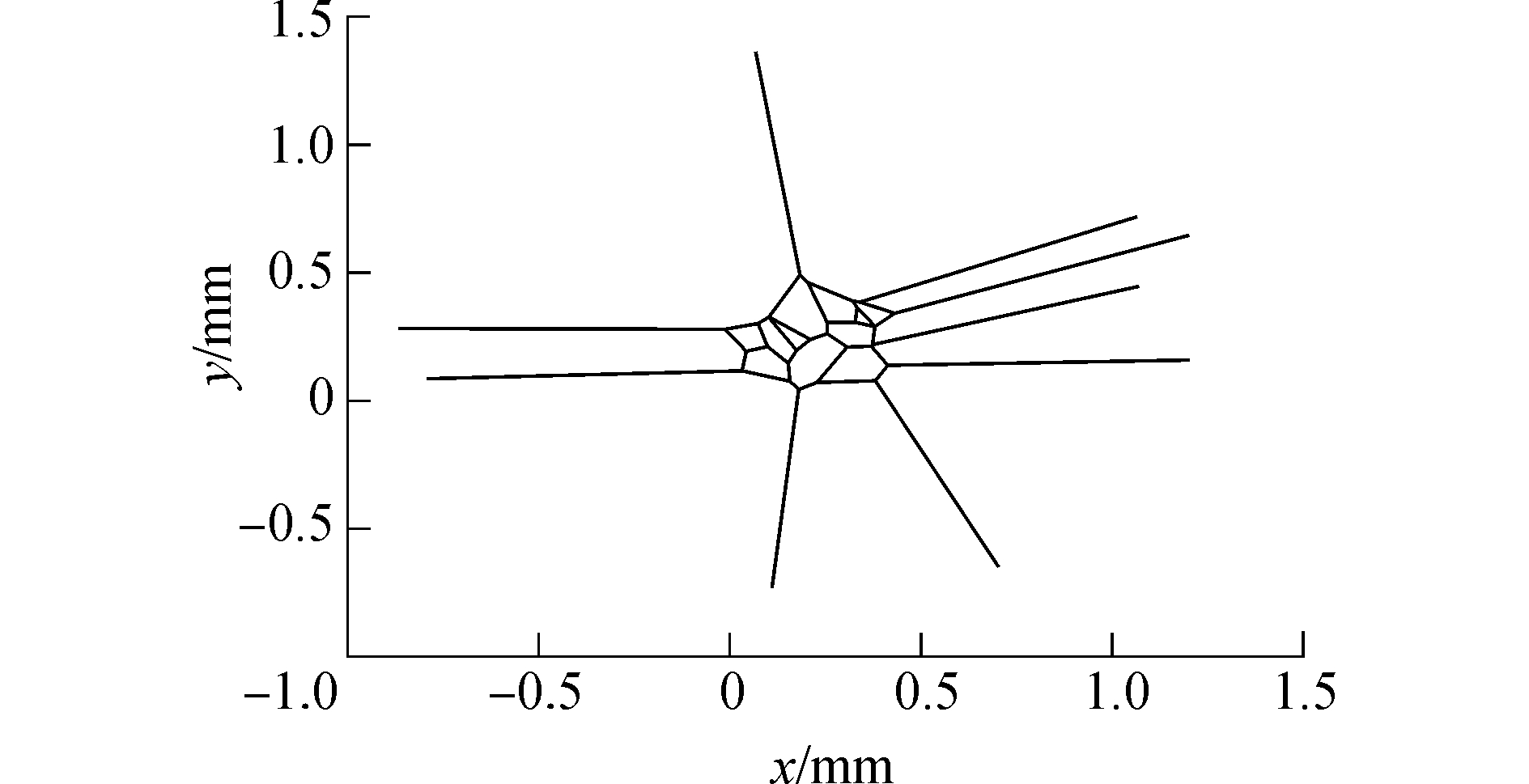Download: 图 1 非限制性voronoi图 Fig. 1 The unrestricted voronoi diagram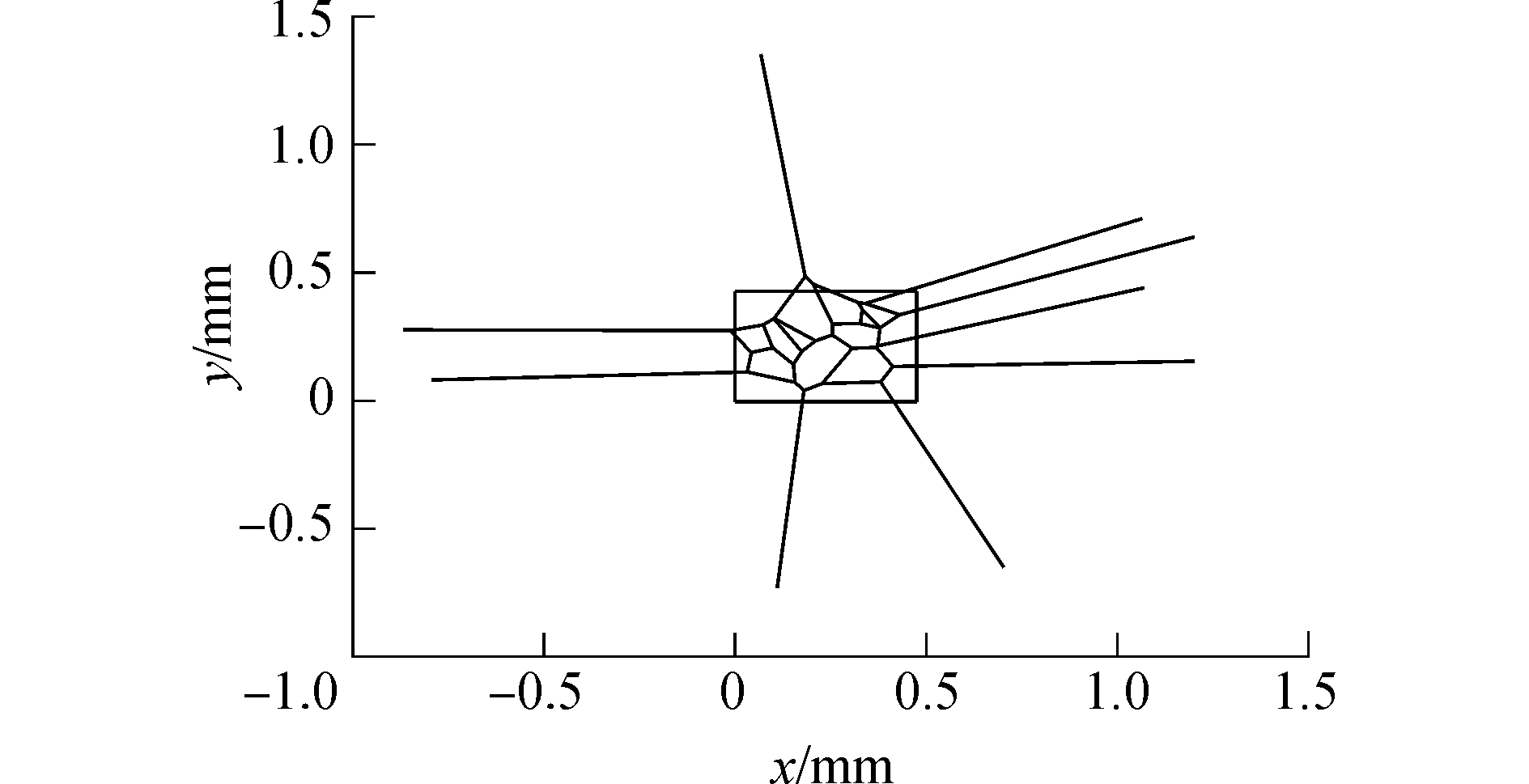Download: 图 2 voronoi图与边界 Fig. 2 The voronoi diagram and its periphery
1.2 限制性voronoi图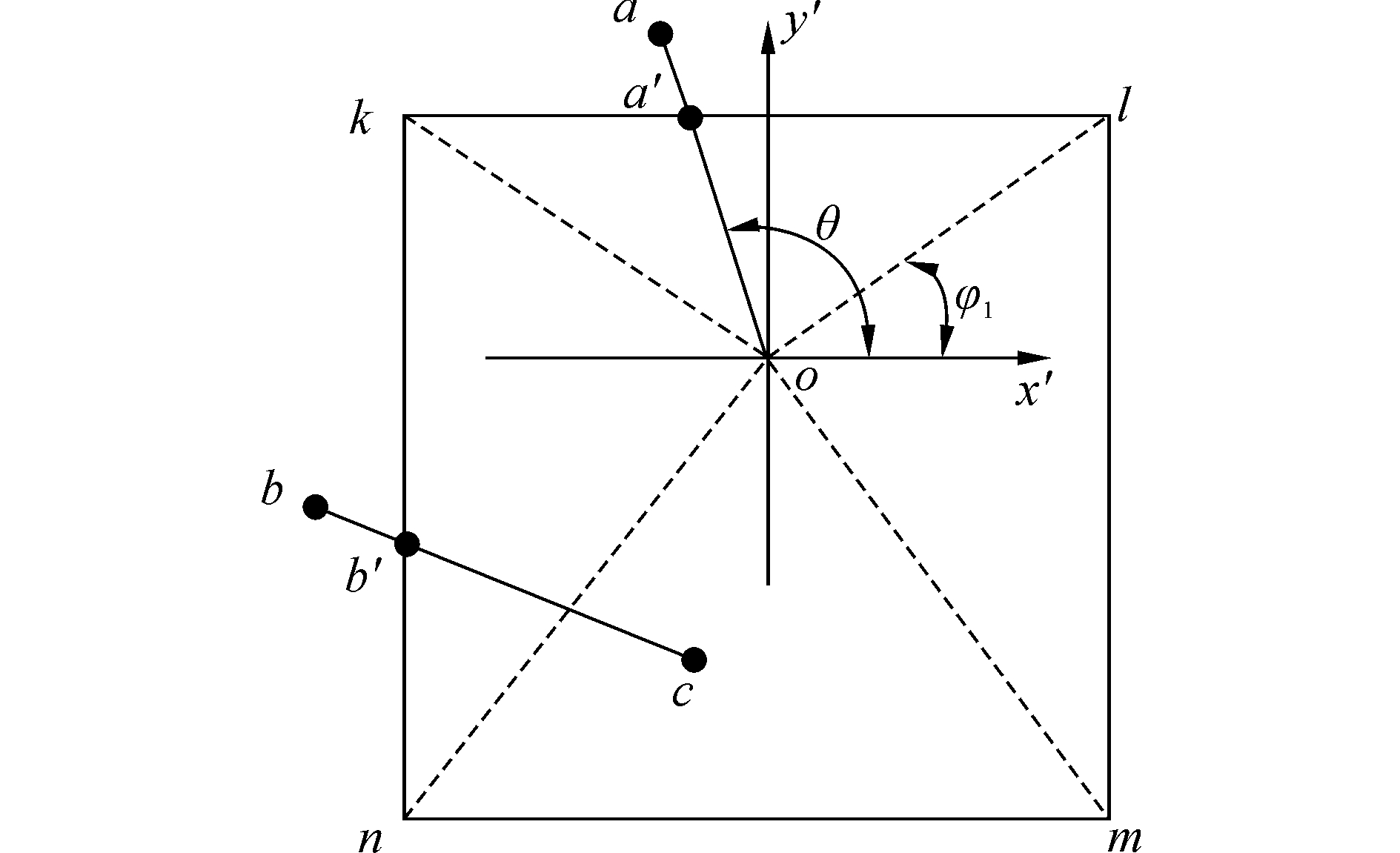Download: 图 3 非限制性voronoi图的剪裁 Fig. 3 The cutting of the unrestricted voronoi diagram

 ${{x'}_a} = {x_o} + \left( {{y_k} - {y_o}} \right){\rm{c}}\tan \theta$ (2)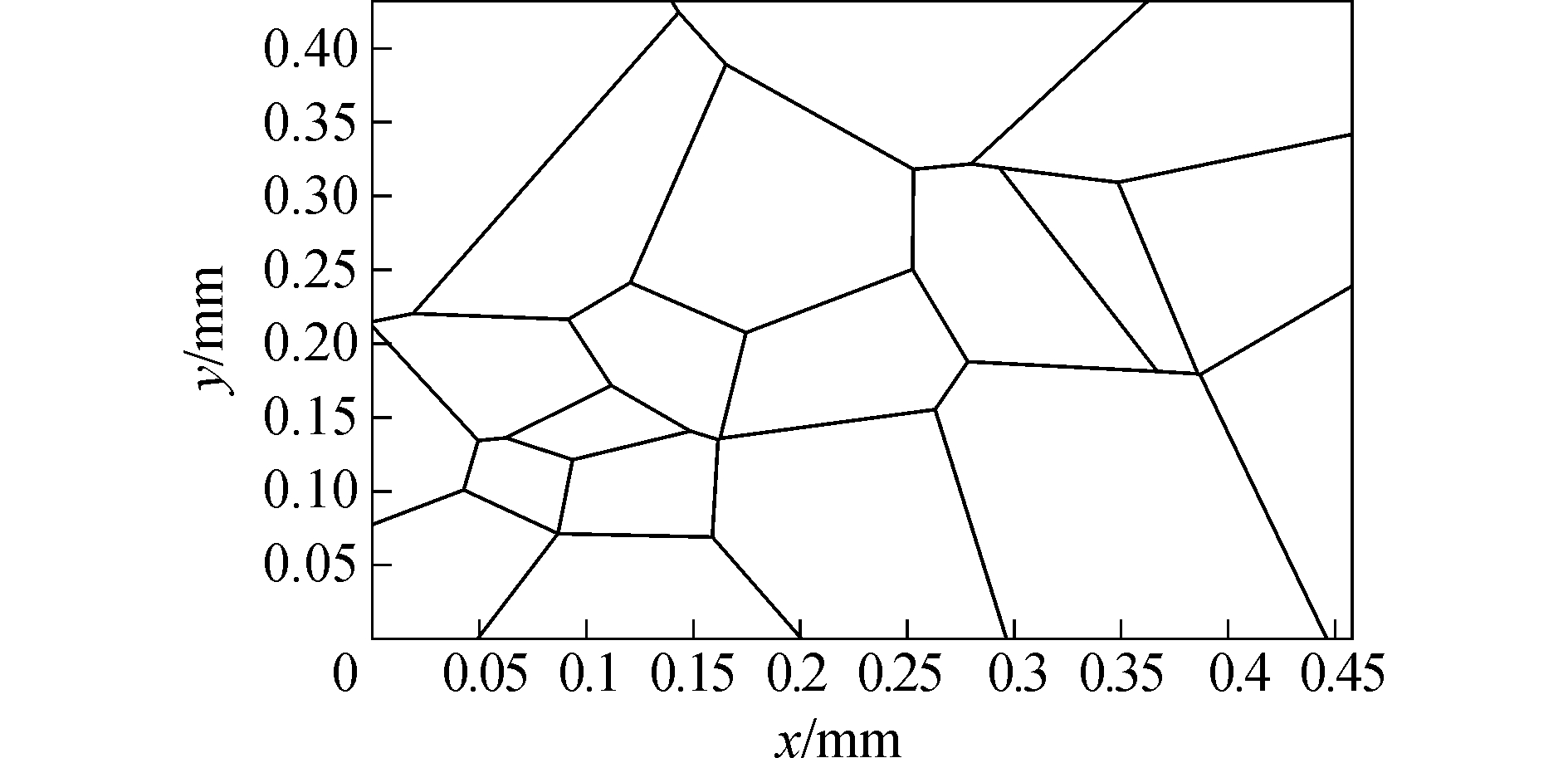Download: 图 4 限制性voronoi图 Fig. 4 The restricted voronoi diagram
2 晶粒的生成

voronoi图被直线分割成很多区域，直观上，每个区域似乎代表一个晶粒，但这只是表面现象。程序生成voronoi图时，并没有把属于同一多边形(晶粒)的直线归在一起，因此在数据结构层次上，这些线段之间没有逻辑联系，为此还要做另一项重要工作，就是把每个晶粒(多边形)所包含的晶界(线段)找到，并存储在一起。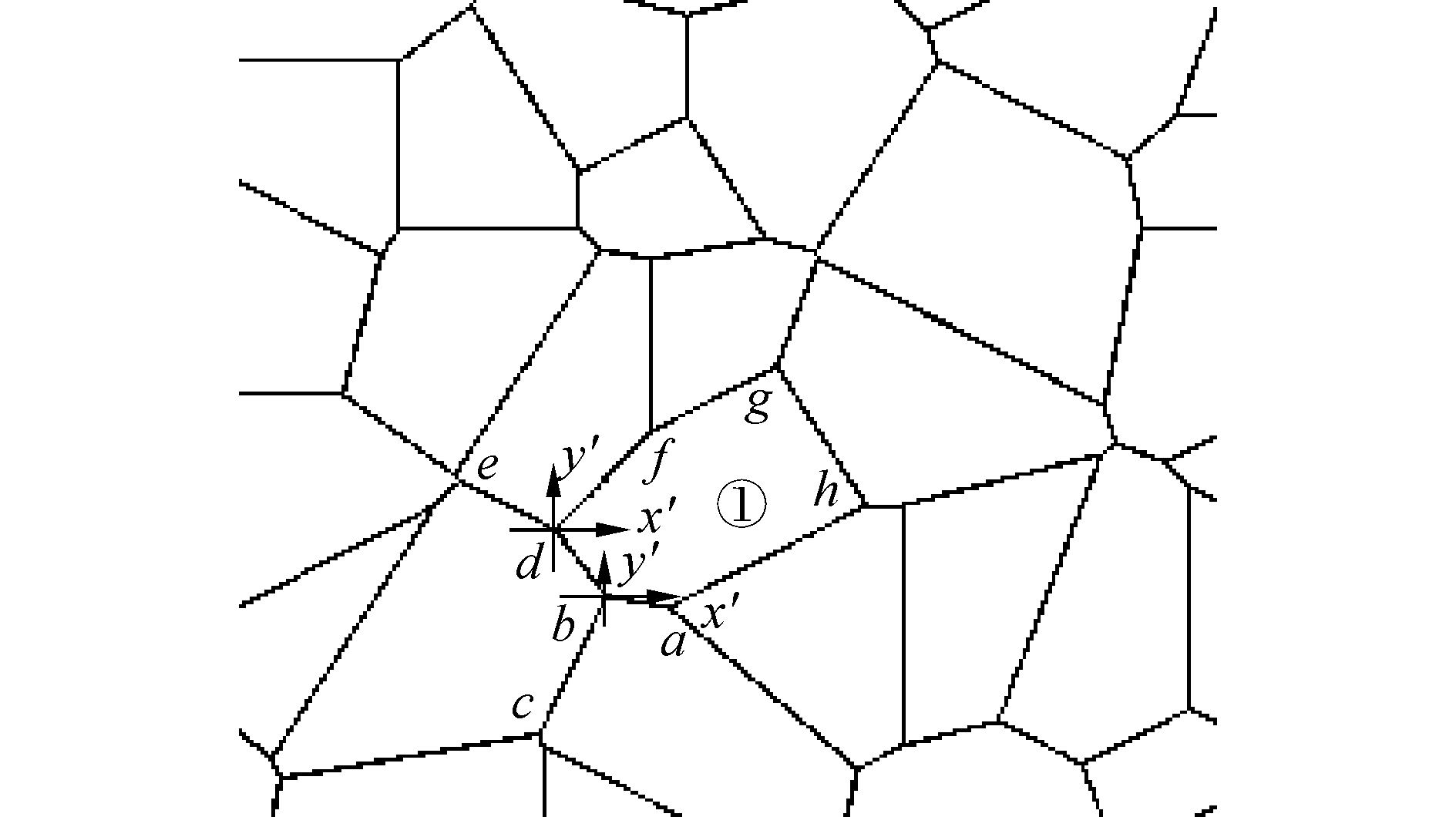Download: 图 5 晶粒的生成 Fig. 5 The generation of a crystal grain

1) 首先选择任一条边，(如ab)作为开始，先将这条边的始点a和终点b的坐标添加到poly中：

 ${\rm{poly}}.\;{\rm{coor}}\left( {1,{\rm{count}}} \right) = {x_a}$
 ${\rm{poly}}.\;{\rm{coor}}\left( {2,{\rm{count}}} \right) = {y_a}$
 ${\rm{poly}}.\;{\rm{coor}}\left( {1,{\rm{count}} + {\rm{1}}} \right) = {x_b}$
 ${\rm{poly}}.\;{\rm{coor}}\left( {2,{\rm{count}} + {\rm{2}}} \right) = {y_b}$

2) 接下来在b点选择下一条属于多边形①的边。因为从b点有两条出发线段的bdbc，必须选择线段bd而舍弃bc。为了能做到这一点，需在b点建立一个如图 5所示的局部坐标系ybx′，然后计算bdbcbx′轴正方向所成的夹角θdθc(图中未标出，可参考图 3中的θ的定义)，然后按顺时针方向进行搜索，也就是说沿着多边形①的边界行走时，多边形始终在行走方向的右侧。

 ${\rm{poly}}.\;{\rm{coor}}\left( {1,{\rm{count}} + {\rm{2}}} \right) = {x_d}$
 ${\rm{poly}}.\;{\rm{coor}}\left( {2,{\rm{count}} + {\rm{2}}} \right) = {y_d}$

3) 接下来，把bd边当成ab边，重复上述过程，就会搜索到f点, 将f点信息记录到poly结构中：

 ${\rm{poly}}.\;{\rm{coor}}\left( {1,{\rm{count}} + {\rm{3}}} \right) = {x_f}$
 ${\rm{poly}}.\;{\rm{coor}}\left( {2,{\rm{count}} + {\rm{3}}} \right) = {y_f}$

4) 在上述搜索过程中，每搜索到新的线段，都需要将新线段的终点(如df线段的f点)的坐标与最初起始点的坐标(即xaya)相比较，如果xf=xayf=ya，则说明线段首尾相接，形成闭合多边形，至此搜索结束，否则继续搜索。当搜索到一个完整的多边形后，就可以把poly添加到结构poly_find中：poly_find (n)=poly，n为多边形(晶粒)序号。

3 计算实例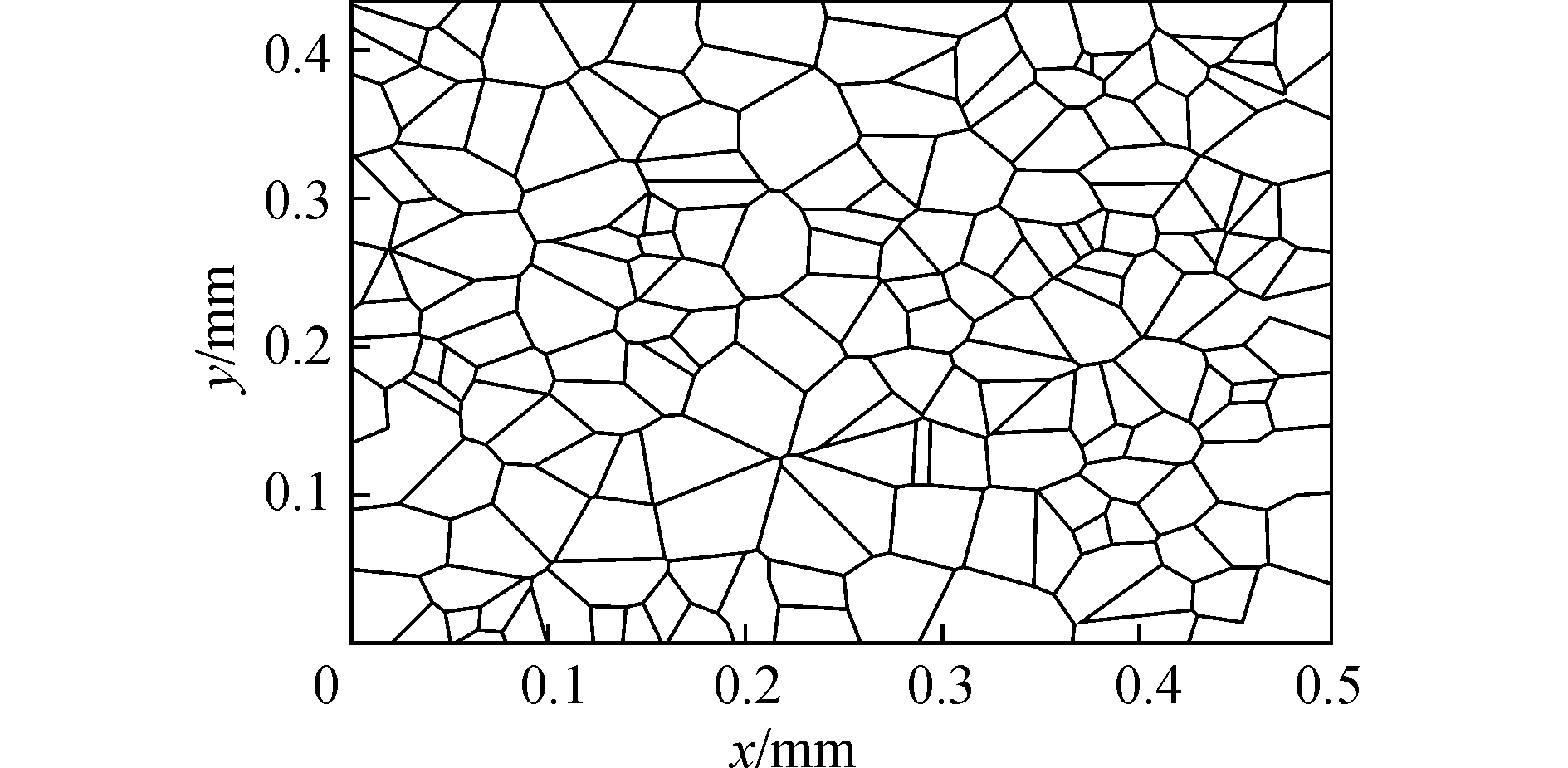Download: 图 6 初始组织 Fig. 6 The initial materials microstructure
3.1 晶核数目的估算

 $I = {I_0}\exp \left( { - \frac{{16{\rm{ \mathsf{ π} }}{\sigma ^3}}}{{3\Delta G_v^2KT}}} \right) \cdot \exp \left( { - \frac{Q}{{KT}}} \right)$ (3)

 ${G_m} = \frac{{\Delta {H_m}}}{{{T_m}}}\Delta T$ (4)

 ${G_V} = \frac{{{G_m}}}{{{V_m}}}$ (5)

Vm为液态铁的摩尔体积, 可由下式得到：

 ${V_m} = \frac{{{M_{F{\rm{e}}}}}}{{{\rho _L}}}$ (6)

 ${\rm{core}}\_{\rm{vol}} = I \times 5.13 \times 35400$ (7)

 ${\rm{core}}\_{\rm{zone}} = 0.5 \times 0.43 \times 1 \times {10^{ - 9}}/{\rm{core}}\_{\rm{vol}} \approx 220\left( {个} \right)$

3.2 初始组织可信度评价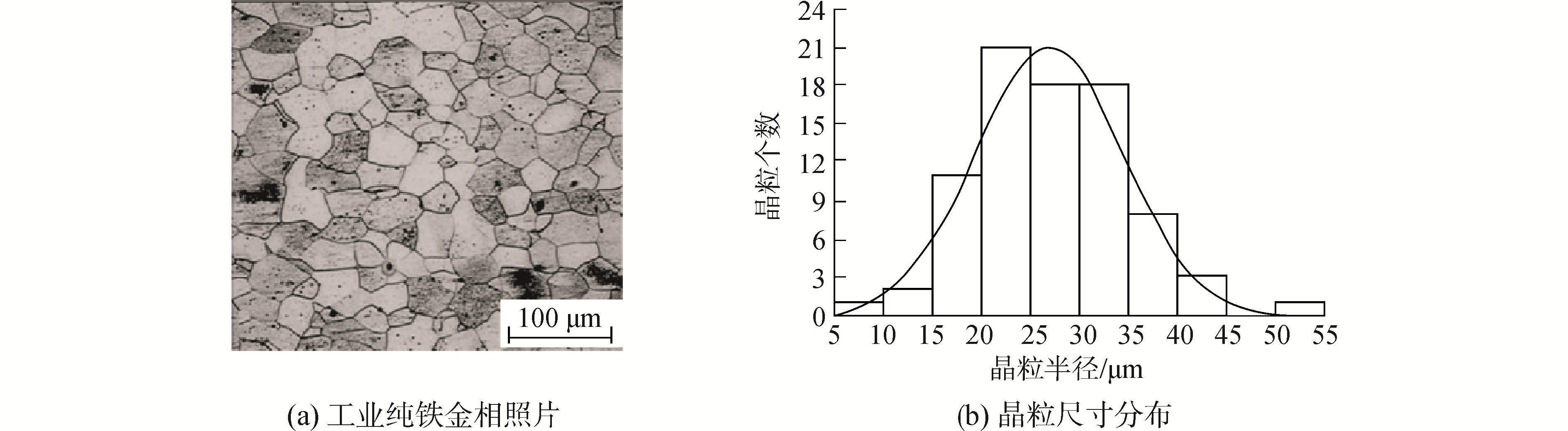Download: 图 7 晶粒尺寸 Fig. 7 Grain size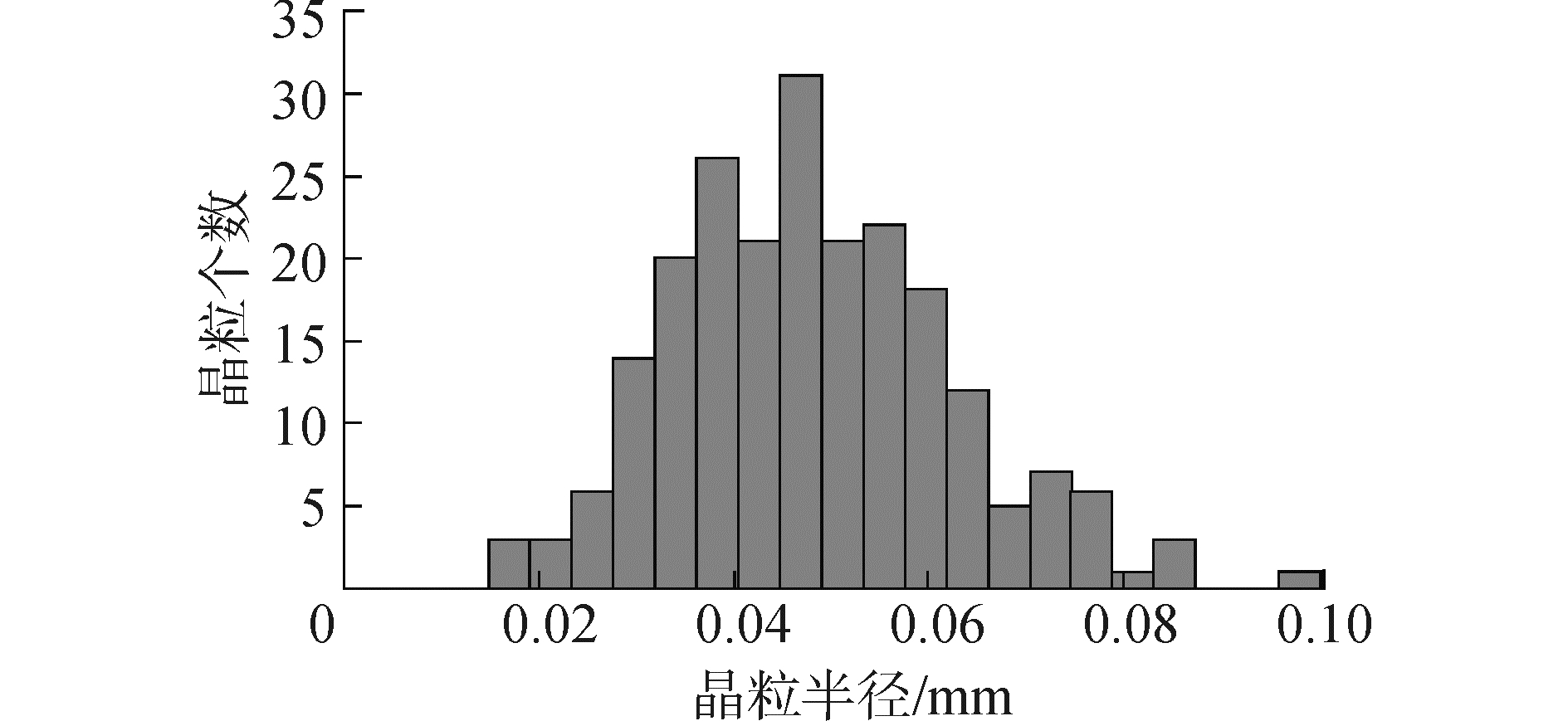Download: 图 8 晶粒尺寸分布 Fig. 8 The distribution of grain size in this study

4 结论

1) 采用本文提出的算法所生成的初始组织，避免了传统方法所产生的微晶现象，比较符合实际情况。

2) 从统计学角度将生成的组织与实际晶粒组织进行了对比，结果非常接近，表明此方法具有一定的可信度。

  ANDERSON M P, SROLOVITZ D J, GREST G S, et al. Computer simulation of grain growth(Ⅰ)-kinetics[J]. Acta metallurgica, 1984, 32(5): 783-791. DOI:10.1016/0001-6160(84)90151-2 (0)  RADHAKRISHNAN B, ZACHARIA T. Simulation of curvature-driven grain growth by using a modified Monte Carlo algorithm[J]. Metallurgical and materials transactions A, 1995, 26(1): 167-180. DOI:10.1007/BF02669802 (0)  MAHIN K W, HANSON K, MORRIS JR W J. Computer simulation of homogeneous nucleation and growth processes[C]//Computer Simulation of Homogeneous Nucleation and Growth Processes. Gaithersburg, MD, USA, 1976: 39-50. https://www.researchgate.net/publication/236365441_Computer_simulation_of_homogeneous_nucleation_and_growth_processes (0)  张继祥, 关小军, 孙胜. 新改进Monte Carlo法模拟钢板退火过程的晶粒长大[J]. 特殊钢, 2004, 25(1): 21-23. ZHANG Jixiang, GUAN Xiaojun, SUN Sheng. A new modified Monte Carlo algorithm for simulation of grain growth of steel plate during annealing[J]. Special steel, 2004, 25(1): 21-23. (0)  莫春立, 李殿中, 钱百年, 等. 铁素体不锈钢焊接热影响区晶粒长大过程模拟[J]. 金属学报, 2001, 37(3): 307-310. MO Chunli, LI Dianzhong, QIAN Bainian, et al. Simulation of grain growth in welding HAZ of ferrite stainless steel[J]. Acta metallurgica sinica, 2001, 37(3): 307-310. (0)  窦晓峰, 鹿守理, 赵辉. 钢静态再结晶的蒙特卡洛模拟[J]. 钢铁研究, 1997(5): 18-21, 26. DOU Xiaofeng, LU Shouli, ZHAO Hui. Monte carol simulation on static recrystallization of steel[J]. Research on iron & steel, 1997(5): 18-21, 26. (0)  方斌, 黄传真, 崇学文, 等. 用改进的Monte Carlo算法模拟晶粒生长[J]. 材料导报, 2008, 22(5): 126-129. FANG Bin, HUANG Chuanzhen, CHONG Xuewen, et al. Simulation of grain Growth with improved Monte Carlo algorithm[J]. Materials review, 2008, 22(5): 126-129. (0)  林清夫. 二相粒子影响静态再结晶过程的数值模拟研究[J]. 材料热处理技术, 2012, 41(14): 86-88. LIN Qingfu. Numerical simulation on effect of second phase particle on static recrystallization[J]. Material & heat treatment, 2012, 41(14): 86-88. (0)  HUMPHREYS F J. A Network model for recovery and recrystallisation[J]. Scripta metallurgica et materialia, 1992, 27(11): 1557-1562. DOI:10.1016/0956-716X(92)90144-4 (0)  DAVIES C H J. The effect of neighbourhood on the kinetics of a cellular automaton recrystallisation model[J]. Scripta metallurgica et materialia, 1995, 33(7): 1139-1143. DOI:10.1016/0956-716X(95)00335-S (0)  MARX V, GOTTSTEIN G. Simulation of the texture evolution of aluminium alloys during primary static recrystallization using a cellular automaton approach[J]. Materials research society symposium proceedings, 1998: 529. DOI:10.1557/PROC-529-107 (0)  刘金义, 刘爽. Voronoi图应用综述[J]. 工程图学学报, 2004(2): 125-132. LIU Jinyi, LIU Shuang. A survey on applications of Voronoi diagrams[J]. Journal of engineering graphics, 2004(2): 125-132. (0)  刘宇雁, 包喜荣, 李振亮, 等. 采用改进的Monte Carlo方法实现晶粒生长的拓扑变化[J]. 安徽工业大学学报, 2005, 22(4): 319-321, 333. LIU Yuyan, BAO Xirong, LI Zhenliang, et al. Using improved Monte Carlo method to realizating the topology change of grain growth[J]. Journal of Anhui University of Technology, 2005, 22(4): 319-321, 333. (0)  钟晓征, 陈伟元, 王豪才, 等. 多晶材料晶粒生长的Monte Carlo计算机模拟方法Ⅰ模拟正常晶粒生长[J]. 功能材料, 1999, 30(3): 232-235. ZHONG Xiaozheng, CHEN Weiyuan, WANG Haocai, et al. Monte Carlo computer methods for simulating grain growth of polycrystalline material Ⅰ Simulating normal grain growth[J]. Functional materials, 1999, 30(3): 232-235. (0)  沈满德, 余圣甫, 王宁. 蒙特卡罗法模拟晶粒生长过程中的Voronoi模型[J]. 机械工程材料, 2006, 30(3): 11-13. SHEN Mande, YU Shengfu, WANG Ning. Voronoi model in Monte Carlo simulation of grain growth[J]. Materials for mechanical engineering, 2006, 30(3): 11-13. (0)  黄诚, 宋波, 毛红, 等. 铁液洁净度对凝固热力学参数的影响[J]. 钢铁研究学报, 2003, 15(4): 9-11, 17. HUANG Cheng, SONG Bo, MAO Hong, et al. Dependence of thermodynamic parameters of solidification on liquid iron cleanness[J]. Journal of iron and steel research, 2003, 15(4): 9-11, 17. (0)  王增辉, 倪明玖. 液态纯铁自扩散系数研究[J]. 工程热物理学报, 2011, 32(8): 1403-1405. WANG Zenghui, NI Mingjiu. Research on self-diffusion coefficient of liquid pure iron[J]. Journal of engineering thermophysics, 2011, 32(8): 1403-1405. (0)  赵亚楠, 郭建政, 李绍敏, 等. 大型钢锭凝固模拟计算中空间与时间步长选取[J]. 铸造, 2013, 62(9): 861-864. ZHAO Yanan, GUO Jianzheng, LI Shaomin, et al. Selection of mesh size and time step in solidification simulation of large ingot[J]. Foundry, 2013, 62(9): 861-864. (0)  朱家明. 基于图像分析软件的晶粒尺寸分布统计[J]. 压电与声光, 2013, 35(4): 585-587. ZHU Jiaming. The measurement of grain size distribution through image analysis software[J]. Piezoelectrics & acoustooptics, 2013, 35(4): 585-587. DOI:10.11977/j.issn.1004-2474.2013.04.030 (0)